Скачать презентацию Chapter 3 Lexical Analysis Definitions The

d349344cf637e83ca2b592d0b67eafb9.ppt

• Количество слайдов: 39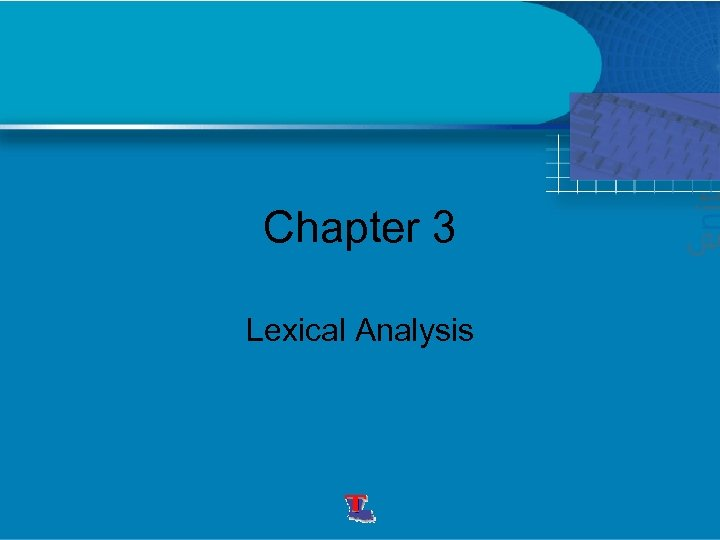Chapter 3 Lexical Analysis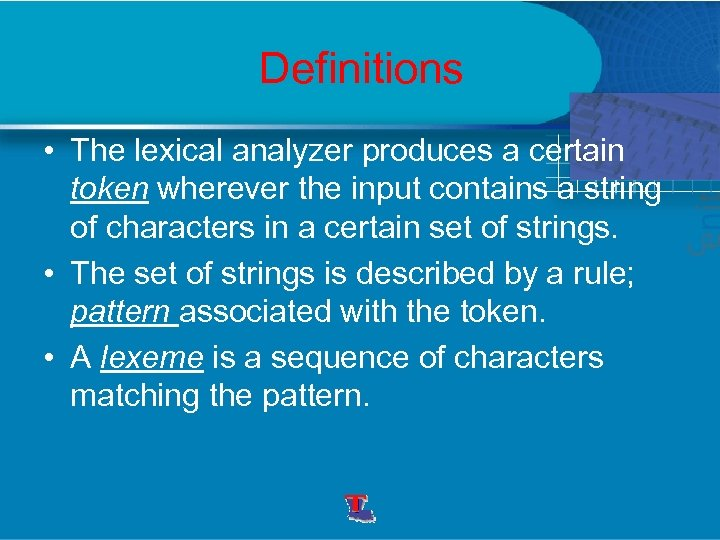Definitions • The lexical analyzer produces a certain token wherever the input contains a string of characters in a certain set of strings. • The set of strings is described by a rule; pattern associated with the token. • A lexeme is a sequence of characters matching the pattern.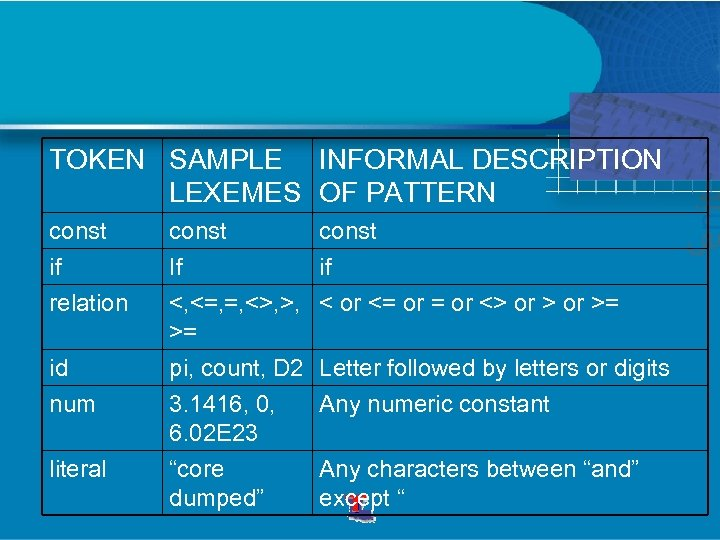TOKEN SAMPLE INFORMAL DESCRIPTION LEXEMES OF PATTERN const if relation id num literal const If <, <=, =, <>, >, >= pi, count, D 2 3. 1416, 0, 6. 02 E 23 const if < or <= or <> or >= “core dumped” Any characters between “and” except “ Letter followed by letters or digits Any numeric constant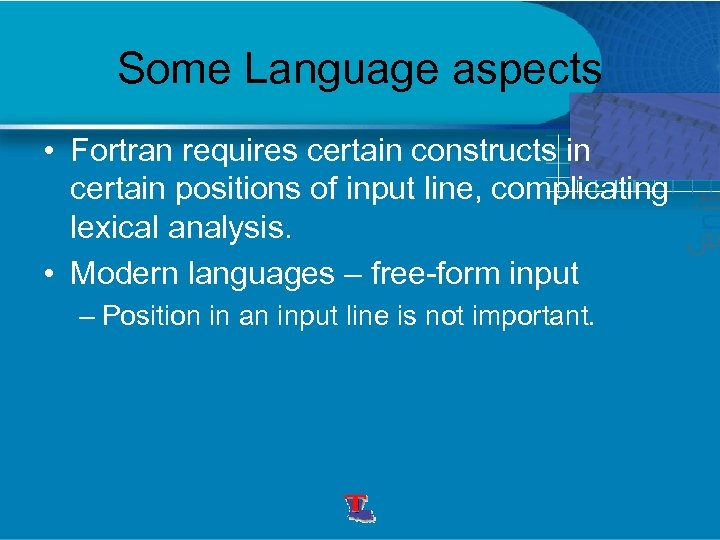Some Language aspects • Fortran requires certain constructs in certain positions of input line, complicating lexical analysis. • Modern languages – free-form input – Position in an input line is not important.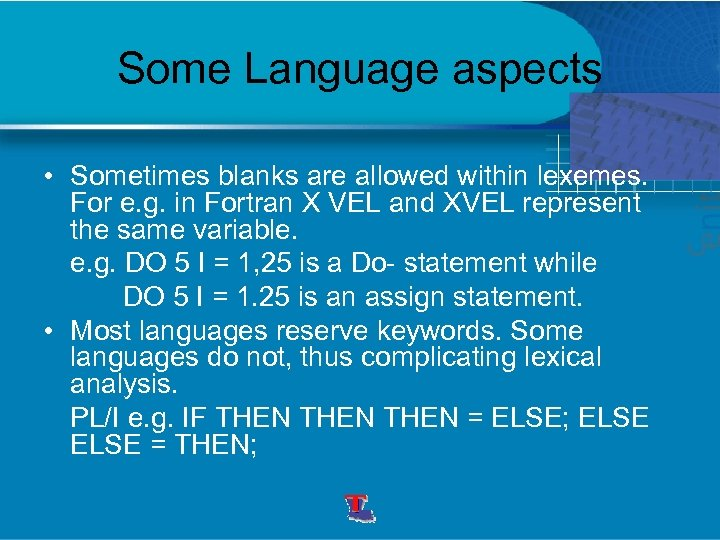Some Language aspects • Sometimes blanks are allowed within lexemes. For e. g. in Fortran X VEL and XVEL represent the same variable. e. g. DO 5 I = 1, 25 is a Do- statement while DO 5 I = 1. 25 is an assign statement. • Most languages reserve keywords. Some languages do not, thus complicating lexical analysis. PL/I e. g. IF THEN = ELSE; ELSE = THEN;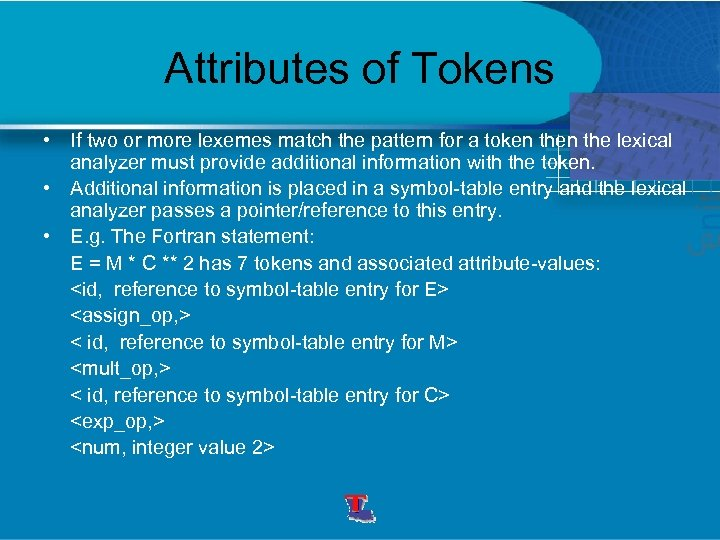Attributes of Tokens • If two or more lexemes match the pattern for a token the lexical analyzer must provide additional information with the token. • Additional information is placed in a symbol-table entry and the lexical analyzer passes a pointer/reference to this entry. • E. g. The Fortran statement: E = M * C ** 2 has 7 tokens and associated attribute-values: < id, reference to symbol-table entry for M> < id, reference to symbol-table entry for C>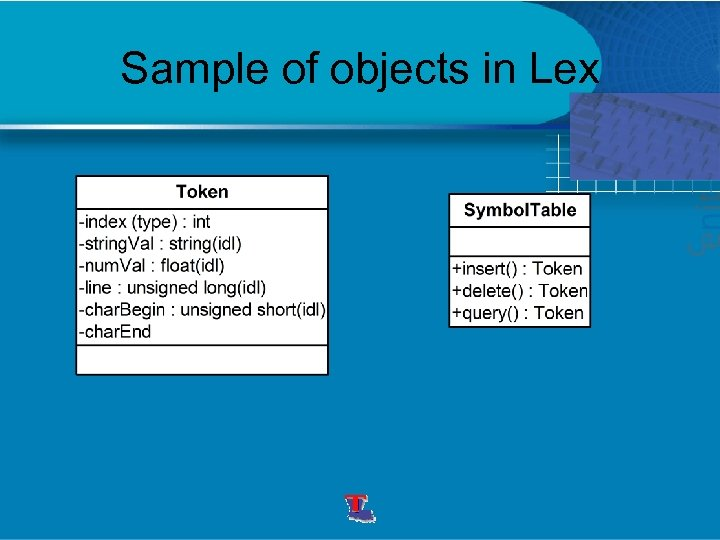Sample of objects in Lex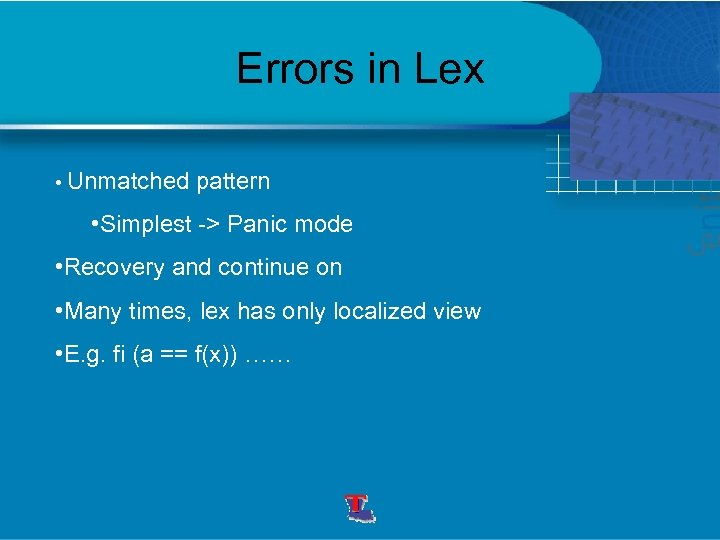Errors in Lex • Unmatched pattern • Simplest -> Panic mode • Recovery and continue on • Many times, lex has only localized view • E. g. fi (a == f(x)) ……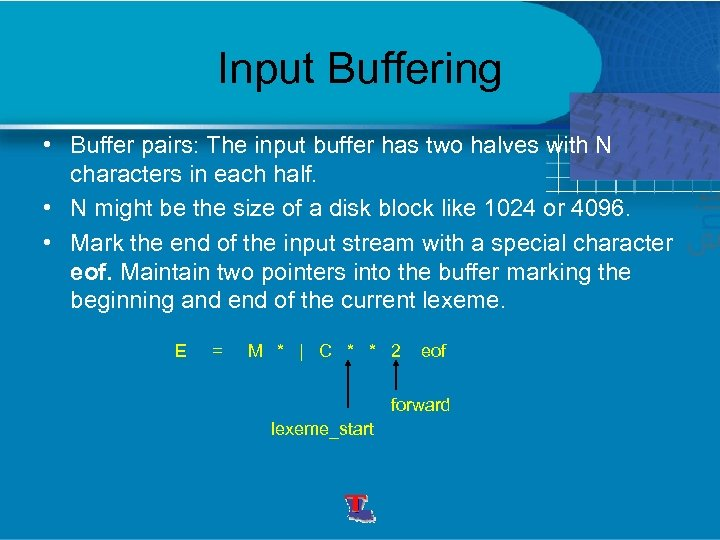Input Buffering • Buffer pairs: The input buffer has two halves with N characters in each half. • N might be the size of a disk block like 1024 or 4096. • Mark the end of the input stream with a special character eof. Maintain two pointers into the buffer marking the beginning and end of the current lexeme. E = M * | C * * 2 eof forward lexeme_start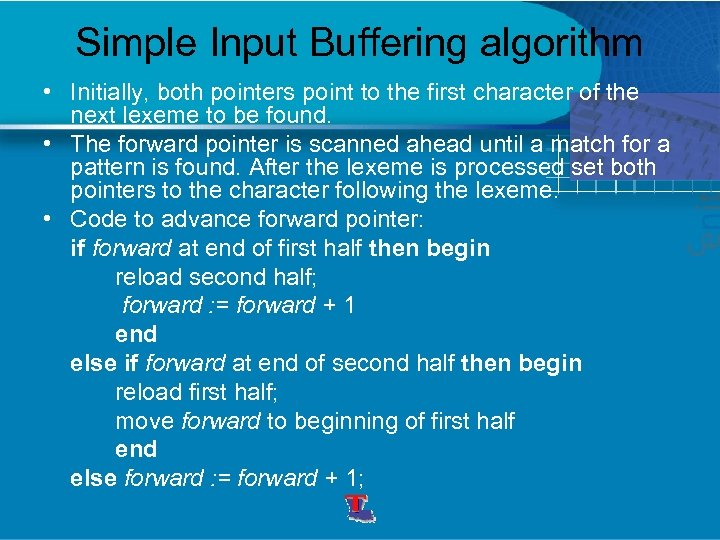Simple Input Buffering algorithm • Initially, both pointers point to the first character of the next lexeme to be found. • The forward pointer is scanned ahead until a match for a pattern is found. After the lexeme is processed set both pointers to the character following the lexeme. • Code to advance forward pointer: if forward at end of first half then begin reload second half; forward : = forward + 1 end else if forward at end of second half then begin reload first half; move forward to beginning of first half end else forward : = forward + 1;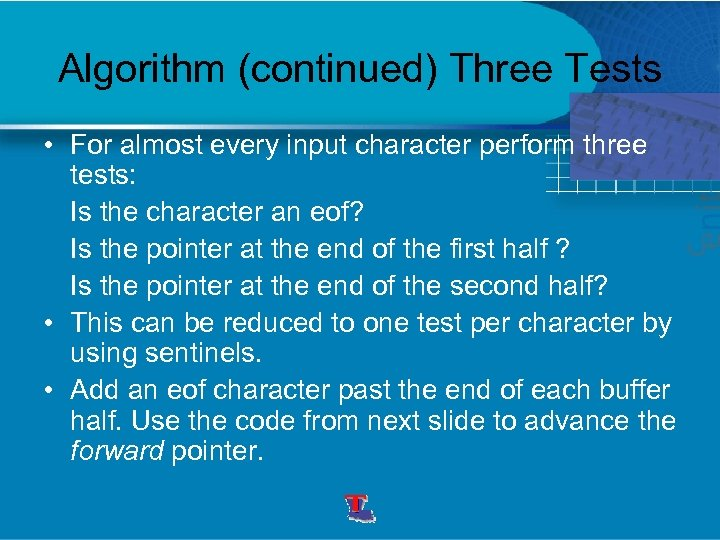Algorithm (continued) Three Tests • For almost every input character perform three tests: Is the character an eof? Is the pointer at the end of the first half ? Is the pointer at the end of the second half? • This can be reduced to one test per character by using sentinels. • Add an eof character past the end of each buffer half. Use the code from next slide to advance the forward pointer.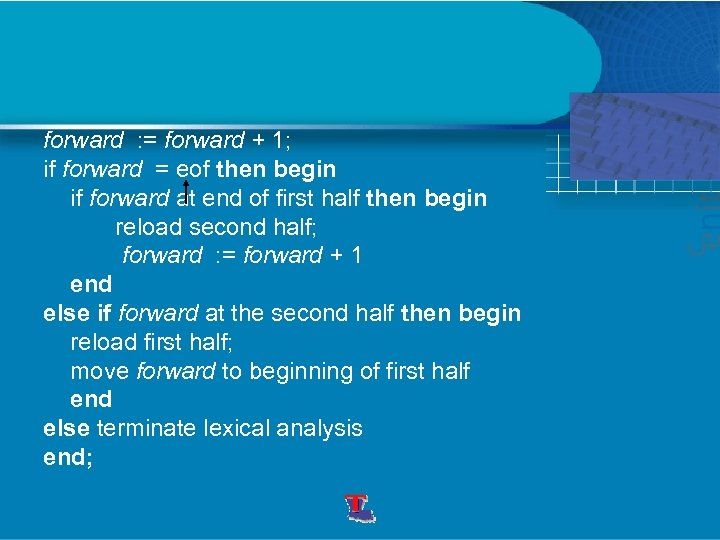forward : = forward + 1; if forward = eof then begin if forward at end of first half then begin reload second half; forward : = forward + 1 end else if forward at the second half then begin reload first half; move forward to beginning of first half end else terminate lexical analysis end;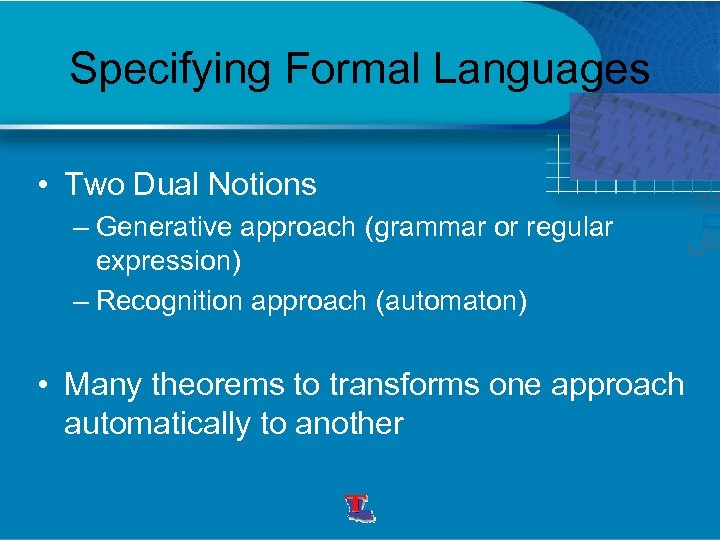Specifying Formal Languages • Two Dual Notions – Generative approach (grammar or regular expression) – Recognition approach (automaton) • Many theorems to transforms one approach automatically to another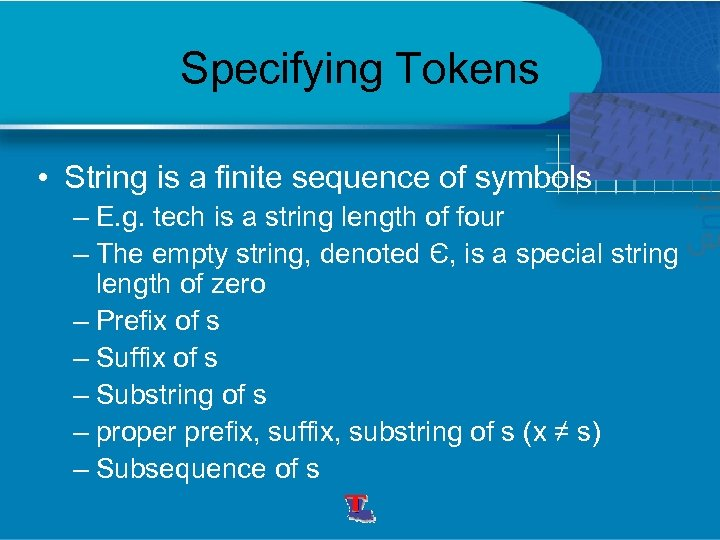Specifying Tokens • String is a finite sequence of symbols – E. g. tech is a string length of four – The empty string, denoted Є, is a special string length of zero – Prefix of s – Suffix of s – Substring of s – proper prefix, suffix, substring of s (x ≠ s) – Subsequence of s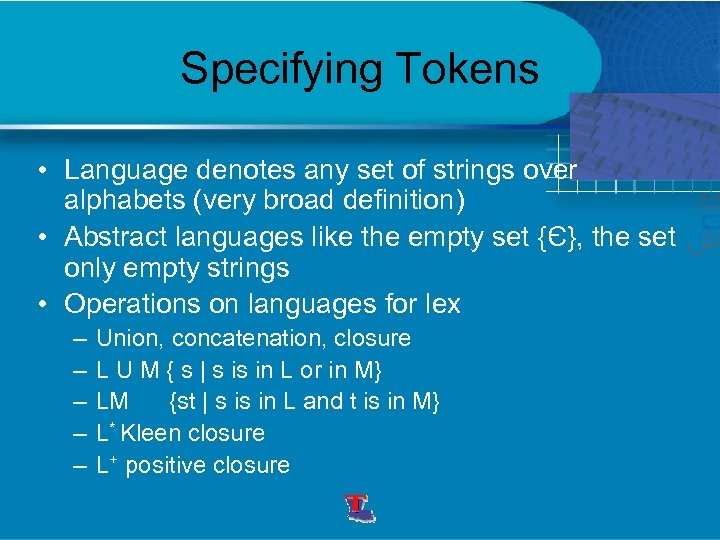Specifying Tokens • Language denotes any set of strings over alphabets (very broad definition) • Abstract languages like the empty set {Є}, the set only empty strings • Operations on languages for lex – – – Union, concatenation, closure L U M { s | s is in L or in M} LM {st | s is in L and t is in M} L* Kleen closure L+ positive closure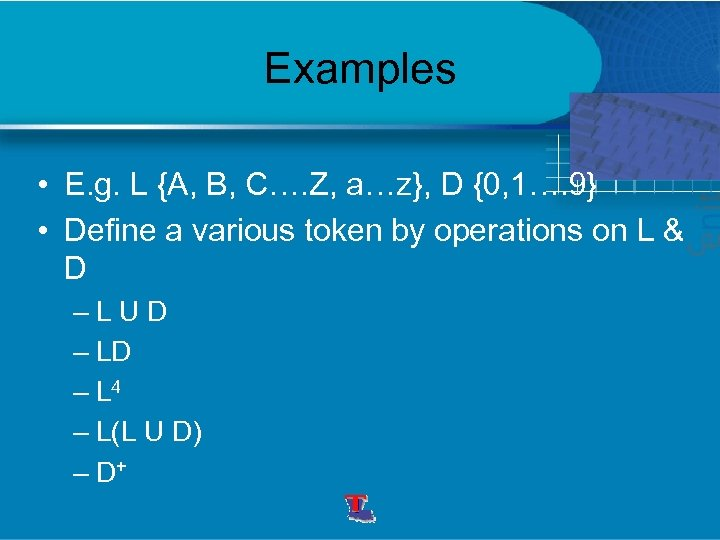Examples • E. g. L {A, B, C…. Z, a…z}, D {0, 1…. 9} • Define a various token by operations on L & D –LUD – L 4 – L(L U D) – D+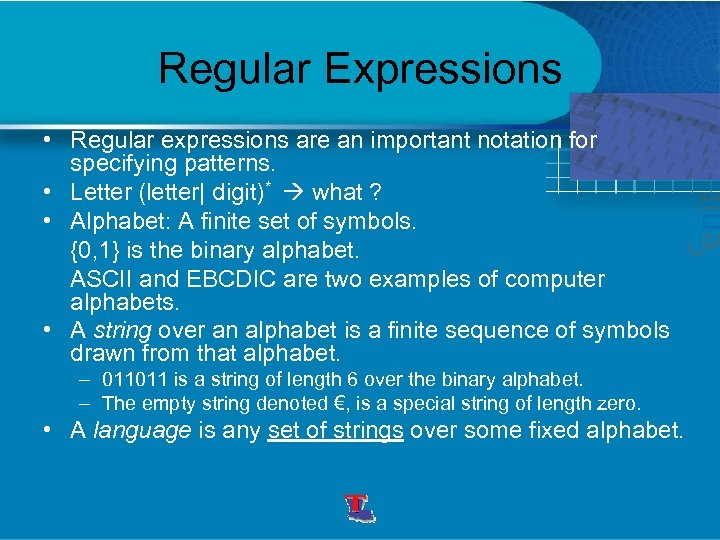Regular Expressions • Regular expressions are an important notation for specifying patterns. • Letter (letter| digit)* what ? • Alphabet: A finite set of symbols. {0, 1} is the binary alphabet. ASCII and EBCDIC are two examples of computer alphabets. • A string over an alphabet is a finite sequence of symbols drawn from that alphabet. – 011011 is a string of length 6 over the binary alphabet. – The empty string denoted €, is a special string of length zero. • A language is any set of strings over some fixed alphabet.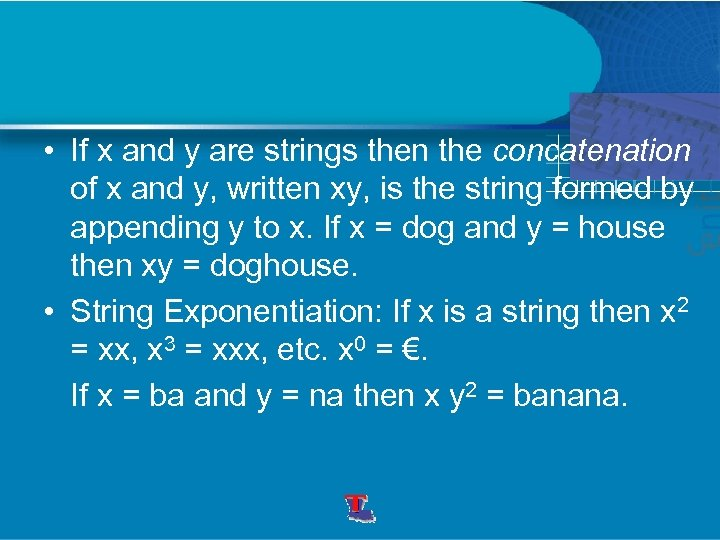• If x and y are strings then the concatenation of x and y, written xy, is the string formed by appending y to x. If x = dog and y = house then xy = doghouse. • String Exponentiation: If x is a string then x 2 = xx, x 3 = xxx, etc. x 0 = €. If x = ba and y = na then x y 2 = banana.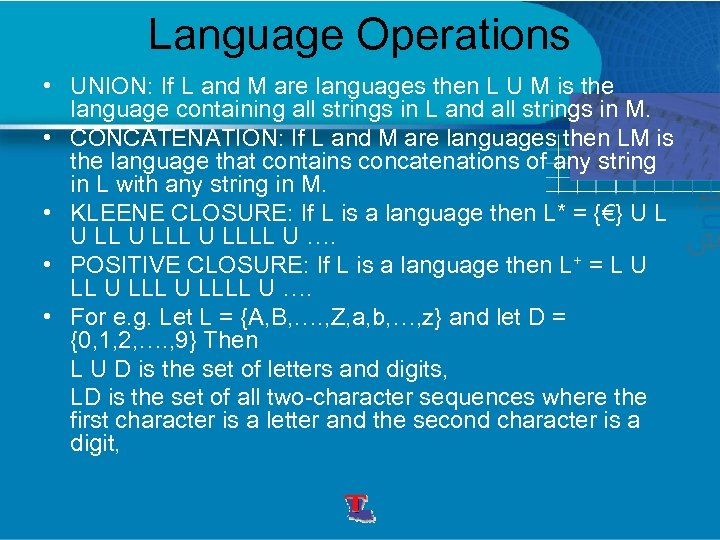Language Operations • UNION: If L and M are languages then L U M is the language containing all strings in L and all strings in M. • CONCATENATION: If L and M are languages then LM is the language that contains concatenations of any string in L with any string in M. • KLEENE CLOSURE: If L is a language then L* = {€} U LLLL U …. • POSITIVE CLOSURE: If L is a language then L+ = L U LLLL U …. • For e. g. Let L = {A, B, …. , Z, a, b, …, z} and let D = {0, 1, 2, …. , 9} Then L U D is the set of letters and digits, LD is the set of all two-character sequences where the first character is a letter and the second character is a digit,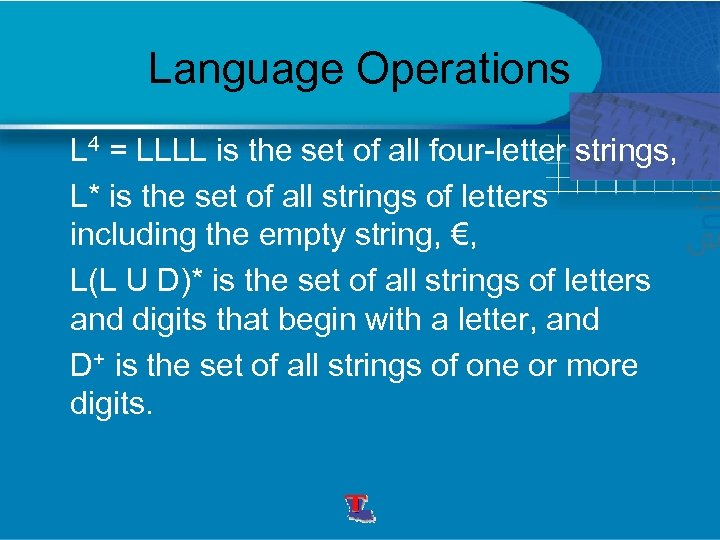Language Operations L 4 = LLLL is the set of all four-letter strings, L* is the set of all strings of letters including the empty string, €, L(L U D)* is the set of all strings of letters and digits that begin with a letter, and D+ is the set of all strings of one or more digits.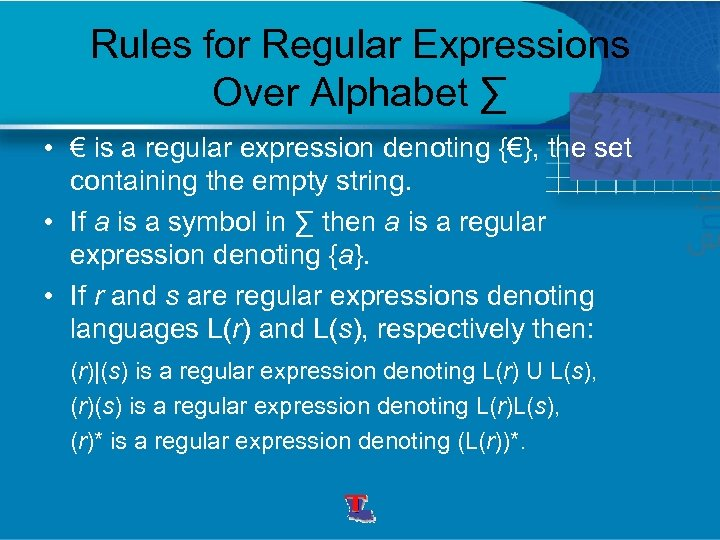Rules for Regular Expressions Over Alphabet ∑ • € is a regular expression denoting {€}, the set containing the empty string. • If a is a symbol in ∑ then a is a regular expression denoting {a}. • If r and s are regular expressions denoting languages L(r) and L(s), respectively then: (r)|(s) is a regular expression denoting L(r) U L(s), (r)(s) is a regular expression denoting L(r)L(s), (r)* is a regular expression denoting (L(r))*.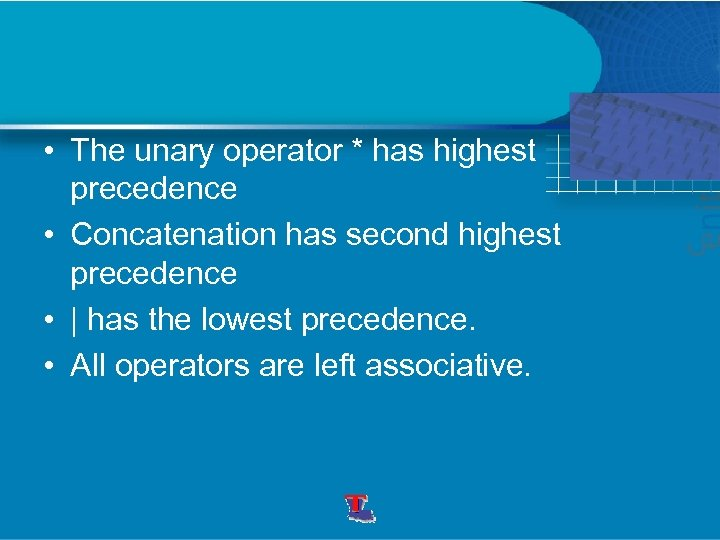• The unary operator * has highest precedence • Concatenation has second highest precedence • | has the lowest precedence. • All operators are left associative.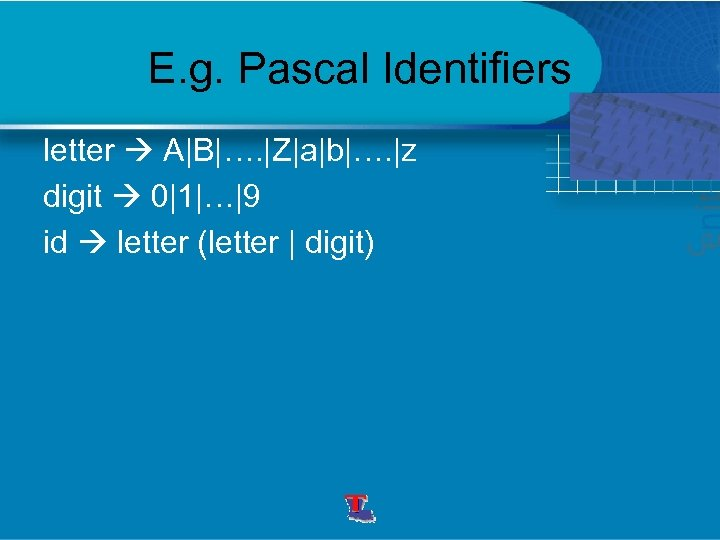E. g. Pascal Identifiers letter A|B|…. |Z|a|b|…. |z digit 0|1|…|9 id letter (letter | digit)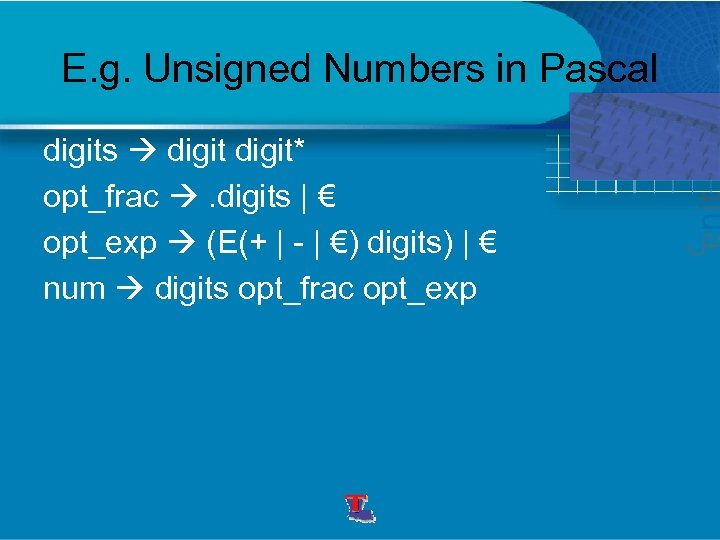E. g. Unsigned Numbers in Pascal digits digit* opt_frac . digits | € opt_exp (E(+ | - | €) digits) | € num digits opt_frac opt_exp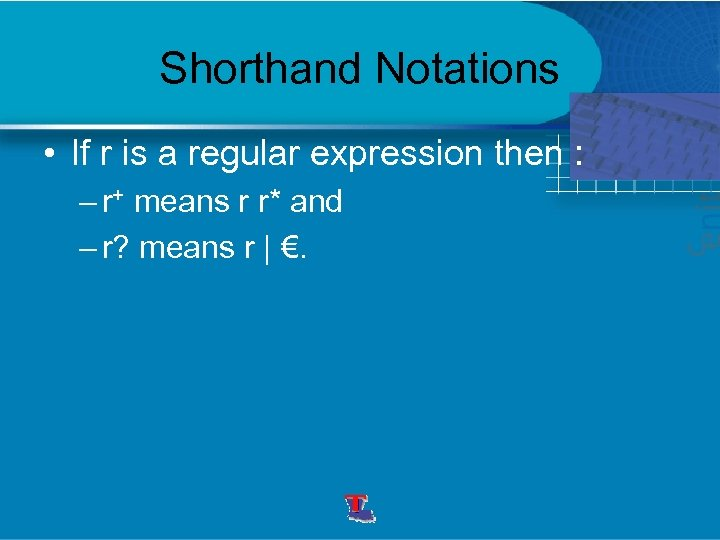Shorthand Notations • If r is a regular expression then : – r+ means r r* and – r? means r | €.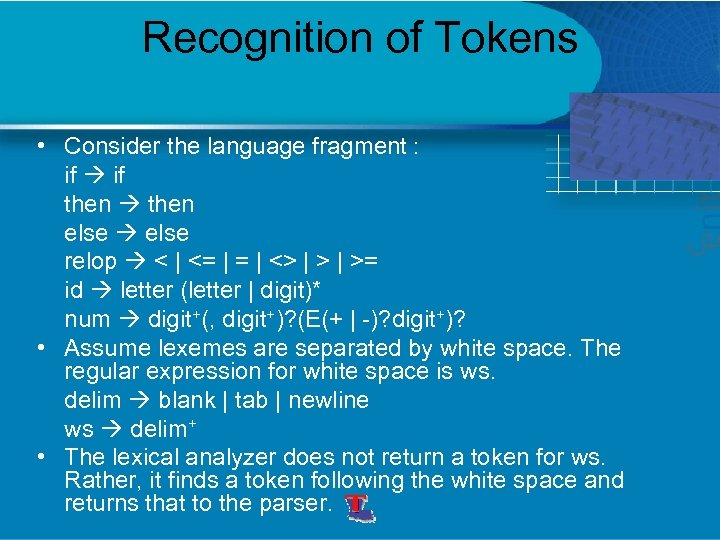Recognition of Tokens • Consider the language fragment : if then else relop < | <= | <> | >= id letter (letter | digit)* num digit+(, digit+)? (E(+ | -)? digit+)? • Assume lexemes are separated by white space. The regular expression for white space is ws. delim blank | tab | newline ws delim+ • The lexical analyzer does not return a token for ws. Rather, it finds a token following the white space and returns that to the parser.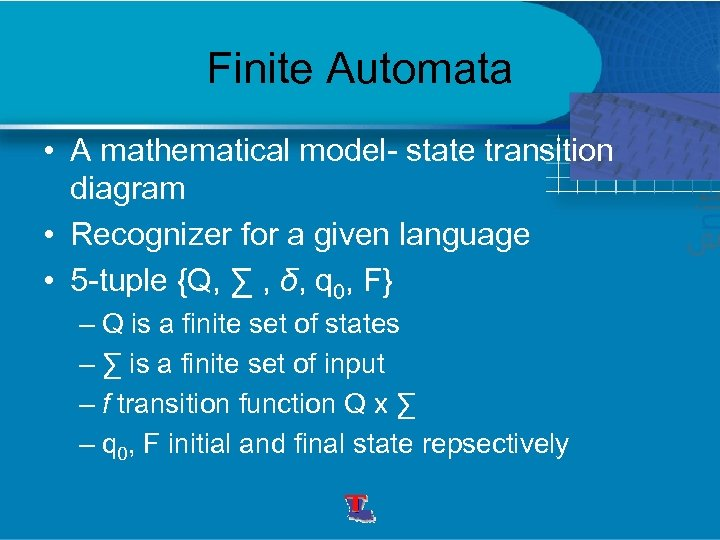Finite Automata • A mathematical model- state transition diagram • Recognizer for a given language • 5 -tuple {Q, ∑ , δ, q 0, F} – Q is a finite set of states – ∑ is a finite set of input – f transition function Q x ∑ – q 0, F initial and final state repsectively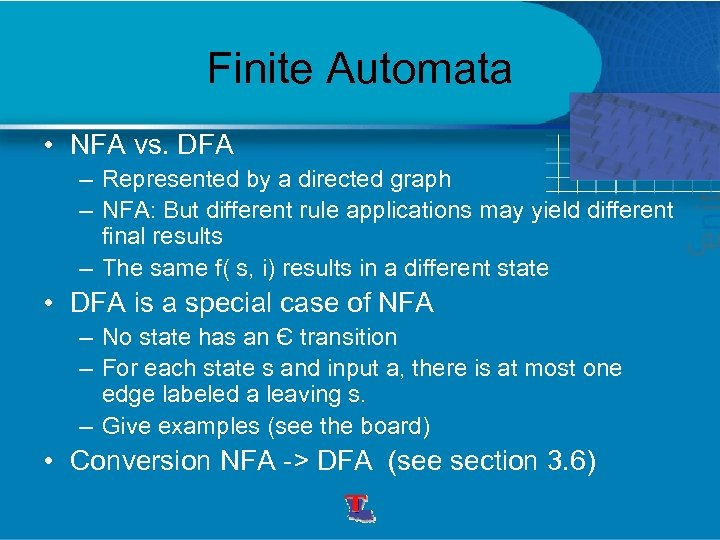Finite Automata • NFA vs. DFA – Represented by a directed graph – NFA: But different rule applications may yield different final results – The same f( s, i) results in a different state • DFA is a special case of NFA – No state has an Є transition – For each state s and input a, there is at most one edge labeled a leaving s. – Give examples (see the board) • Conversion NFA -> DFA (see section 3. 6)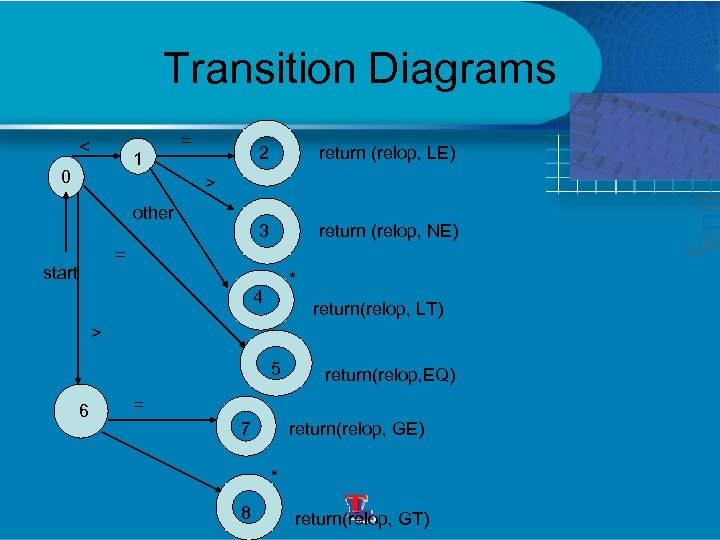Transition Diagrams < 1 0 = 2 return (relop, LE) 3 return (relop, NE) > other = start * 4 return(relop, LT) > 5 6 return(relop, EQ) = 7 return(relop, GE) * 8 return(relop, GT)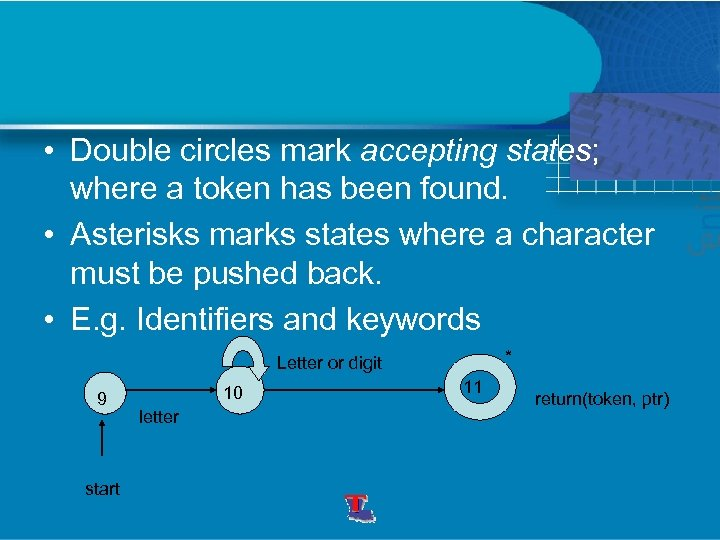• Double circles mark accepting states; where a token has been found. • Asterisks marks states where a character must be pushed back. • E. g. Identifiers and keywords * Letter or digit 9 start 10 letter 11 return(token, ptr)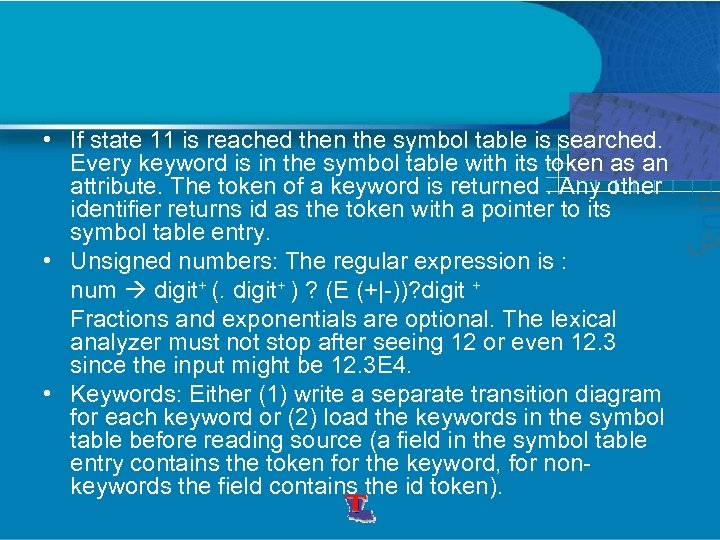• If state 11 is reached then the symbol table is searched. Every keyword is in the symbol table with its token as an attribute. The token of a keyword is returned. Any other identifier returns id as the token with a pointer to its symbol table entry. • Unsigned numbers: The regular expression is : num digit+ (. digit+ ) ? (E (+|-))? digit + Fractions and exponentials are optional. The lexical analyzer must not stop after seeing 12 or even 12. 3 since the input might be 12. 3 E 4. • Keywords: Either (1) write a separate transition diagram for each keyword or (2) load the keywords in the symbol table before reading source (a field in the symbol table entry contains the token for the keyword, for nonkeywords the field contains the id token).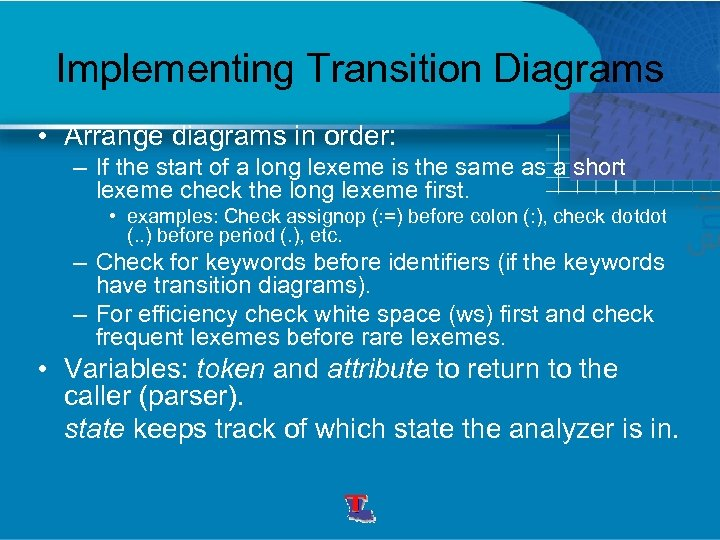Implementing Transition Diagrams • Arrange diagrams in order: – If the start of a long lexeme is the same as a short lexeme check the long lexeme first. • examples: Check assignop (: =) before colon (: ), check dotdot (. . ) before period (. ), etc. – Check for keywords before identifiers (if the keywords have transition diagrams). – For efficiency check white space (ws) first and check frequent lexemes before rare lexemes. • Variables: token and attribute to return to the caller (parser). state keeps track of which state the analyzer is in.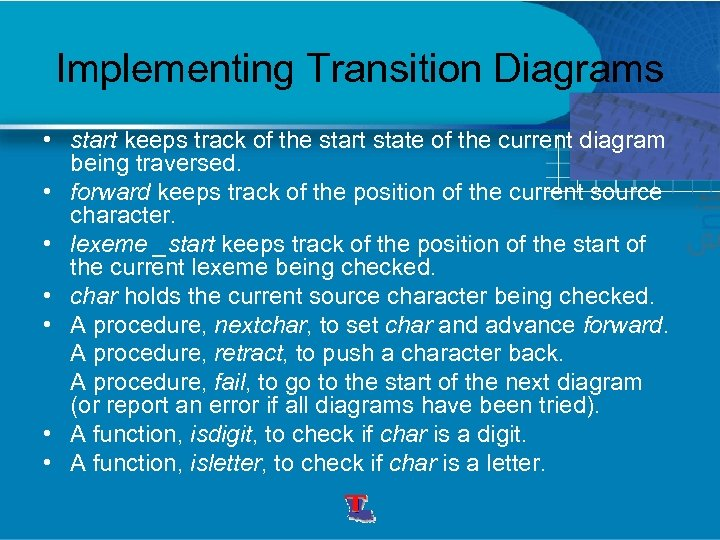Implementing Transition Diagrams • start keeps track of the start state of the current diagram being traversed. • forward keeps track of the position of the current source character. • lexeme _start keeps track of the position of the start of the current lexeme being checked. • char holds the current source character being checked. • A procedure, nextchar, to set char and advance forward. A procedure, retract, to push a character back. A procedure, fail, to go to the start of the next diagram (or report an error if all diagrams have been tried). • A function, isdigit, to check if char is a digit. • A function, isletter, to check if char is a letter.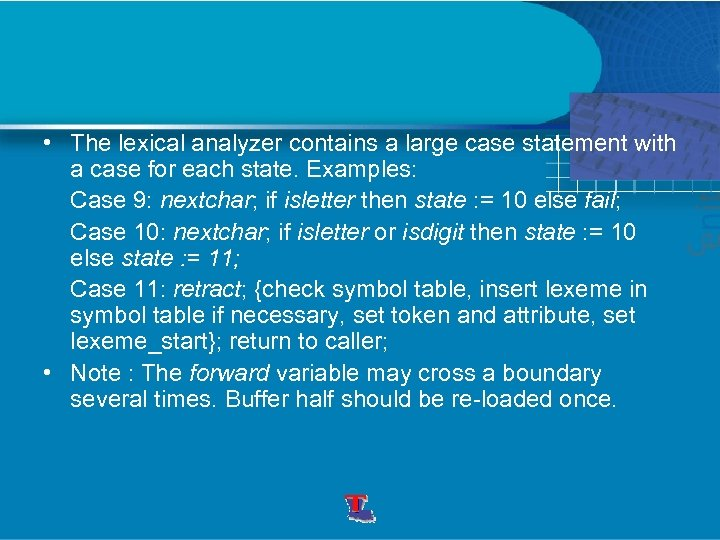• The lexical analyzer contains a large case statement with a case for each state. Examples: Case 9: nextchar; if isletter then state : = 10 else fail; Case 10: nextchar; if isletter or isdigit then state : = 10 else state : = 11; Case 11: retract; {check symbol table, insert lexeme in symbol table if necessary, set token and attribute, set lexeme_start}; return to caller; • Note : The forward variable may cross a boundary several times. Buffer half should be re-loaded once.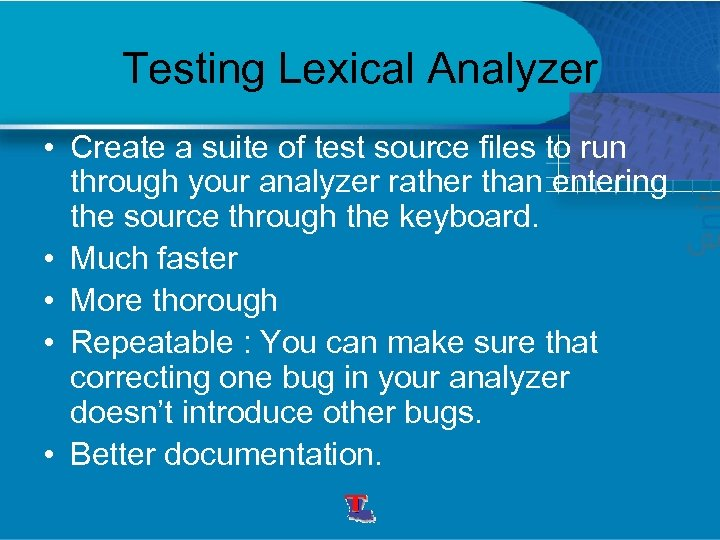Testing Lexical Analyzer • Create a suite of test source files to run through your analyzer rather than entering the source through the keyboard. • Much faster • More thorough • Repeatable : You can make sure that correcting one bug in your analyzer doesn’t introduce other bugs. • Better documentation.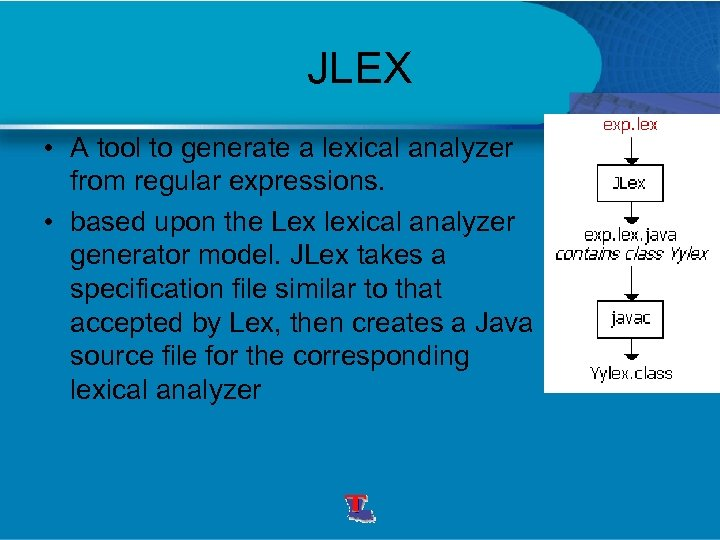JLEX • A tool to generate a lexical analyzer from regular expressions. • based upon the Lex lexical analyzer generator model. JLex takes a specification file similar to that accepted by Lex, then creates a Java source file for the corresponding lexical analyzer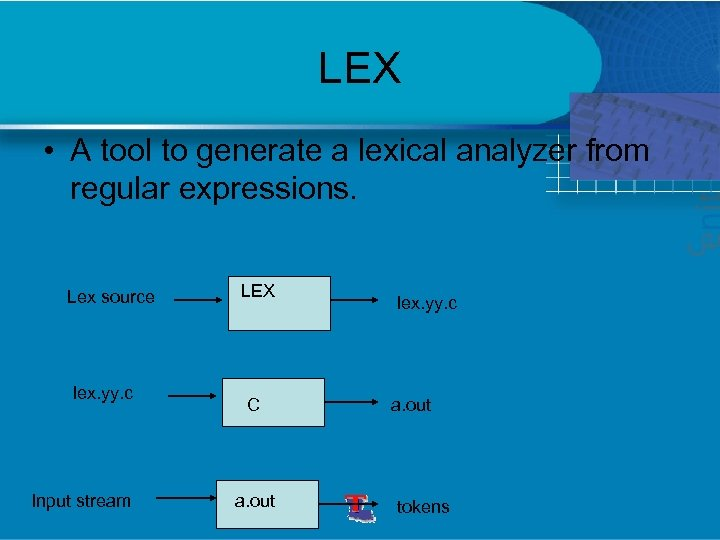LEX • A tool to generate a lexical analyzer from regular expressions. Lex source lex. yy. c Input stream LEX C a. out lex. yy. c a. out tokens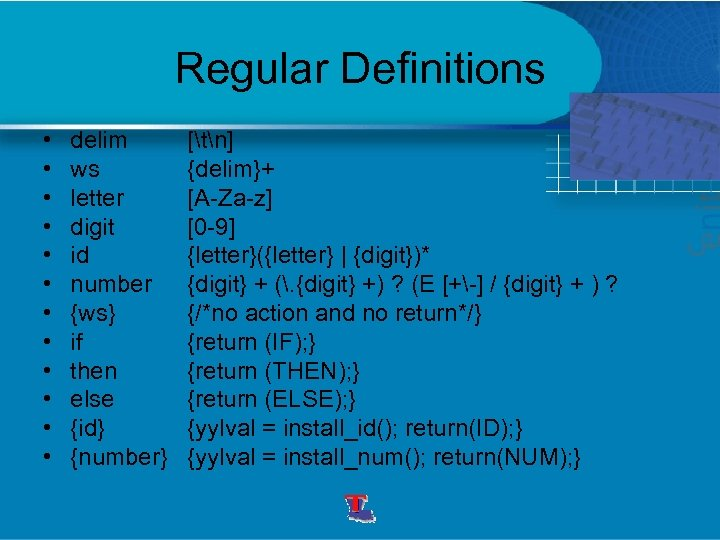Regular Definitions • • • delim ws letter digit id number {ws} if then else {id} {number} [tn] {delim}+ [A-Za-z] [0 -9] {letter}({letter} | {digit})* {digit} + (. {digit} +) ? (E [+-] / {digit} + ) ? {/*no action and no return*/} {return (IF); } {return (THEN); } {return (ELSE); } {yylval = install_id(); return(ID); } {yylval = install_num(); return(NUM); }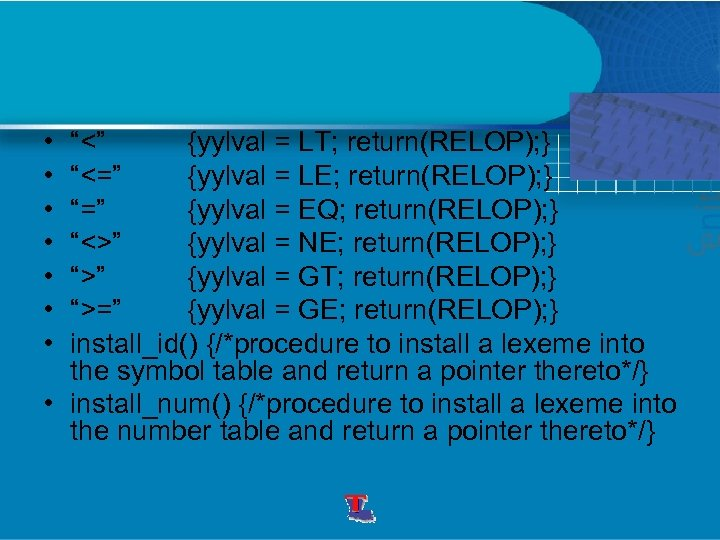• • “<” {yylval = LT; return(RELOP); } “<=” {yylval = LE; return(RELOP); } “=” {yylval = EQ; return(RELOP); } “<>” {yylval = NE; return(RELOP); } “>” {yylval = GT; return(RELOP); } “>=” {yylval = GE; return(RELOP); } install_id() {/*procedure to install a lexeme into the symbol table and return a pointer thereto*/} • install_num() {/*procedure to install a lexeme into the number table and return a pointer thereto*/}